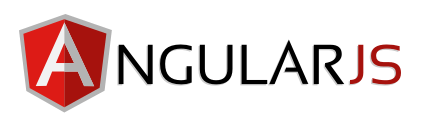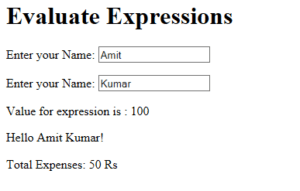# angularJS expression example

AngularJS expressions are JavaScript-like code snippets used to bind application data to html.  The syntax for the Expressions uses  double braces like {{ expression}} or {{ 1+1 }} . The below given are the valid expressions in the angularJS script execution

• `5+5`
• `x+y`
• `user.name`
• `products[index]`

The below sample shows the usage of different ways of executing expressions in angularJS

Sample code:

<html>
<body>
<h1>Evaluate Expressions</h1>
<div ng-app = “” ng-init = “quantity = 5;cost = 10;”>
<p>Enter First Name: <input type = “text” ng-model = “firstName”></p>
<p>Enter Last Name: <input type = “text” ng-model = “lastName”></p>

<p>Value for  expression is : {{ 50 + 50 }}</p>
<p>Hello {{firstName + ” ” + lastName}}!</p>
<p>Total Expenses: {{cost * quantity}} Rs</p>

</div>
</body>
</html># angularJS bind input variable

The blog shows the simple example of how to bind the input variables in the angularJS .

The ng-bind directive  binds the values of AngularJS application data to HTML input controls.

Example

```<div ng-app = "">
...
</div>```

Sample Code:

<html>